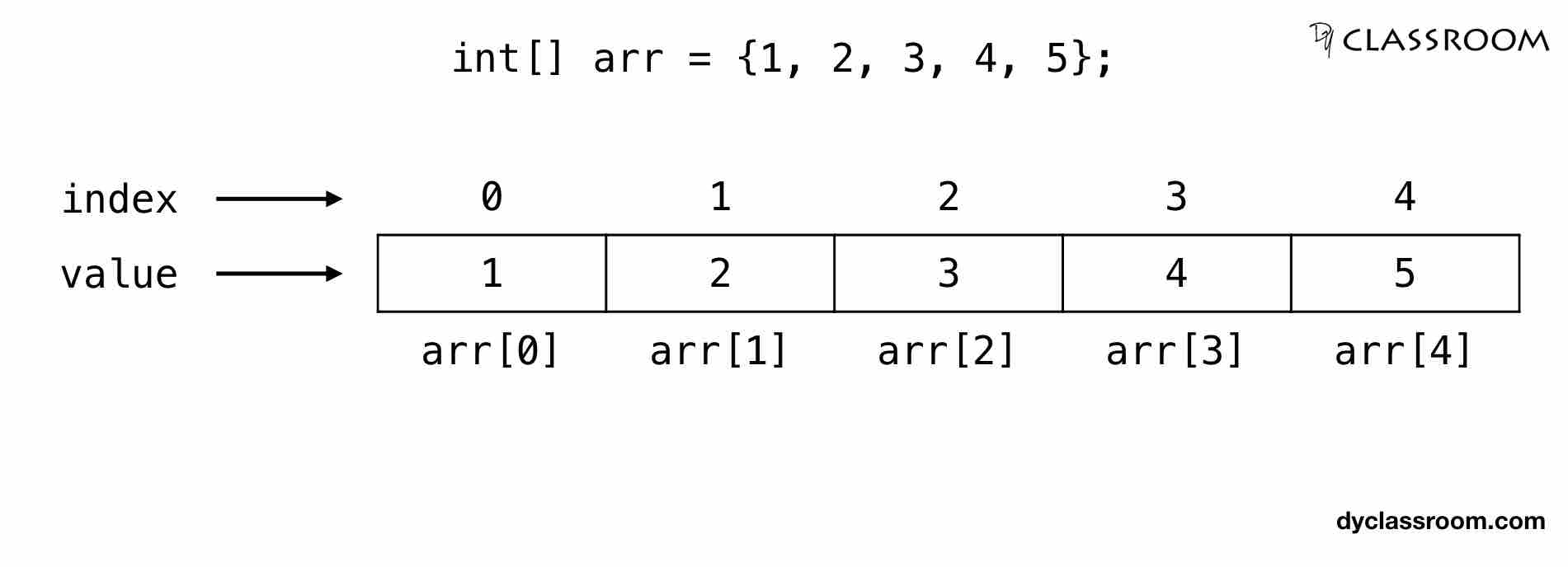# Java - Arrays

JavaIn this tutorial we will learn about Arrays in Java programming language.

## What is an Array?

An array is a fixed size variable to store one or more values of a given data type.

The items stored in the array are called the elements of the array.

The elements are accessed by their array index which starts from 0.

So, if an array has 3 elements then the first element is at index 0, the second element is at index 1 and the last element is at index 2.

We create array to store similar data type values under one variable name. So, if we are required to save 10 integer values then we can create either ten integer variables or one array variable of type integer.

## Types of Array

We generally divide arrays in three categories.

• One dimensional array or 1D array
• Two dimensional array or 2D array
• Multi dimensional arrays like 3D array and above

In this tutorial we will cover 1D array. And in the next part we will cover the 2D array.

## Declaring a 1D array

Following is the syntax to declare a 1D array.

``````dataType[] arrayName;
``````

Where, `dateType` is the data type of the array variable having name `arrayName`.

We can also declare 1D array in the following manner.

``````dataType arrayName[];
``````

This is similar to C programming language.

In the following example we are declaring a 1D array `arr` of data type `int` to store integer values.

``````int[] arr;
``````

We have declared an array variable but we have not yet created it. By creation we mean allocating memory space to the array variable. So, lets go ahead and create a 1D array.

## Creating a 1D array

To create an array we use the `new` keyword as shown below.

``````arrayName = new dataType[size];
``````

Where, `arrayName` is the name of the array variable of data type `dataType` and of given `size`.

In the following example we are creating an array `arr` of size 5 to hold five elements of data type `int`.

``````arr = new int;
``````

## Declaration and Creation of 1D array

We can merge the declaration and creation steps of an array into one step.

In the following example we are declaring and creating a 1D array `arr` of size 5 to hold five values of data type `int`.

``````int[] arr = new int;
``````

## Assigning and Accessing value of a 1D array

In the following example we are creating a 1D array of size 5 and assigning integer values using for loop.

To access the elements of the array we are using the array name and index of the element like `arr[i]` where, i is an index.

``````class ArrayExample {
public static void main(String args[]) {
// create an array
int[] arr = new int;

// use for loop to assign value to an array
for (int i = 0; i < 5; i++) {
arr[i] = i+1;
}

// print the value of the array
for (int i = 0; i < 5; i++) {
System.out.println("arr[" + i + "] = " + arr[i]);
}
}
}
``````

Output:

``````arr = 1
arr = 2
arr = 3
arr = 4
arr = 5
``````

Another way of assigning the values is by using `{}` curly brackets and using comman separated values.

``````class ArrayExample {
public static void main(String args[]) {
// create an array
int[] arr = {1, 2, 3, 4, 5};

// print the value of the array
for (int i = 0; i < 5; i++) {
System.out.println("arr[" + i + "] = " + arr[i]);
}
}
}
``````

We can even assign value index-wise like the following.

``````class ArrayExample {
public static void main(String args[]) {
// create an array
int[] arr = new int;

// assign value
arr = 1;
arr = 2;
arr = 3;
arr = 4;
arr = 5;

// print the value of the array
for (int i = 0; i < 5; i++) {
System.out.println("arr[" + i + "] = " + arr[i]);
}
}
}
``````

## Representation of 1D array

The following image depicts a 1D array `arr`.

``````int[] arr = {1, 2, 3, 4, 5};
``````## Example #1: Write a program in Java to create a 1D array of integer type to store score of 5 students and find the average

Given, marks of the 5 students are 70, 82, 50, 45 and 91.

So, we will create an array `score` of type `int` to store the score of the five students.

We will also create a variable `sum` of type `int` to hold the sum of the score and variable `avg` of type `float` to hold the average.

Hint: avg = sum/n

Where, avg is the average, sum is the total of all the elements and n is the number of elements.

Check out Average tutorial for more details.

``````class ArrayExample {
public static void main(String args[]) {
// create an array
int[] arr = {70, 82, 50, 45, 91};

// int variable
int sum = 0;

// float variable
float avg = 0;

// compute sum
for (int i = 0; i < 5; i++) {
sum += arr[i];
}

// find average
avg = (float)sum / 5;

// print result
System.out.println("Average score = " + avg);
}
}
``````

Output:

``````Average score = 67.6
``````

## Example #2: Write a program in Java to compute the speed of a train in kph if it covers 100km in 48 minutes

Hint: S = D / T

Where, S = speed, D = distance and T = time

Check out Speed Distance and Time tutorial for more details.